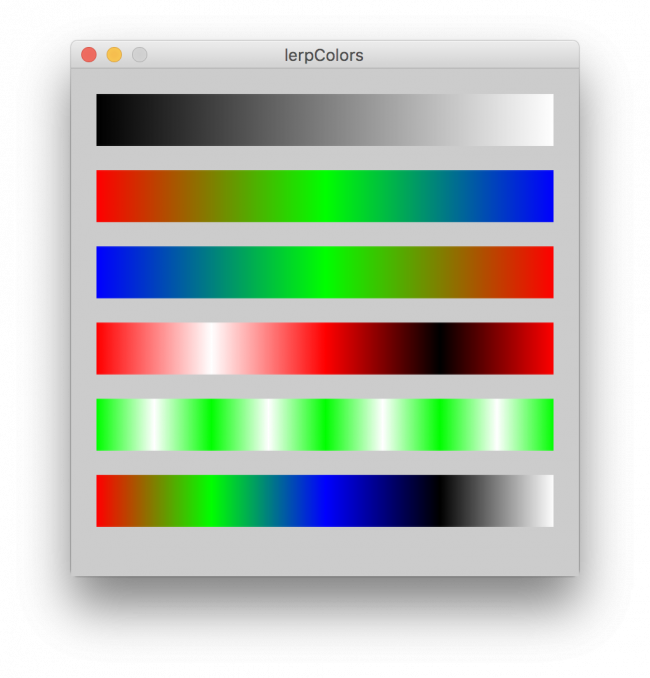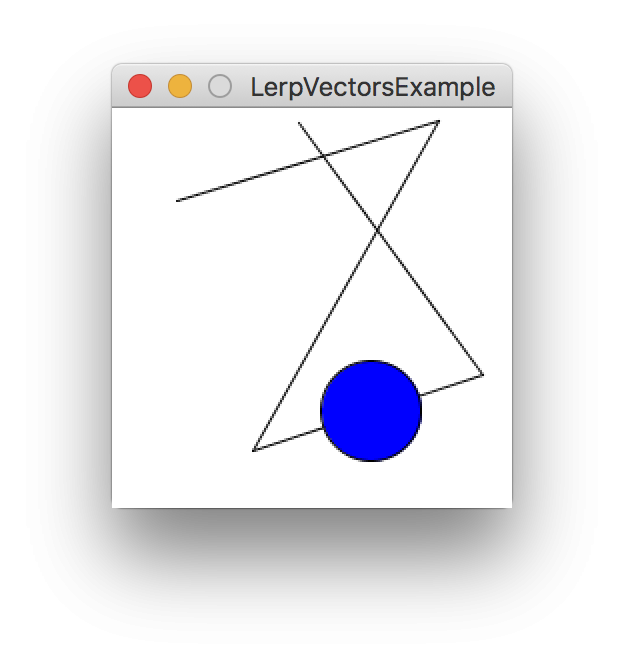#### Howdy, Stranger!

We are about to switch to a new forum software. Until then we have removed the registration on this forum.

# List-based interpolations

edited March 2018

I've been thinking about array-based interpolations recently.

One that often comes up is how to interpolate not just between two colors, but across an arbitrarily long list of three or more colors, e.g. a heatmap scale blue-white-red:

``````color lerpColors(float amt, color... colors) {
if(colors.length==1){ return colors; }
float unit = 1.0/(colors.length-1);
return lerpColor(colors[floor(amt / unit)], colors[ceil(amt / unit)], amt%unit/unit);
}
``````

Another that I see is complex path navigation: how to interpolate not just between two PVectors, but along an arbitrarily long list of three or more points, e.g. a sprite patrolling path:

``````PVector lerpVectors(float amt, PVector... vecs) {
if(vecs.length==1){ return vecs; }
float unit = 1.0/(vecs.length-1);
return PVector.lerp(vecs[floor(amt / unit)], vecs[ceil(amt / unit)], amt%unit/unit);
}
``````

Of course, this can be done when interpolating multiple floats as well:

``````float lerps( float amt, float... vals) {
if(vals.length==1){ return vals; }
float unit = 1.0/(vals.length-1);
return lerp(vals[floor(amt / unit)], vals[ceil(amt / unit)], amt%unit/unit);
}
``````

These could also be implemented for ArrayLists rather than arrays.

This problem seems fairly fundamental to the idea of interpolation, and comes up a lot. I wonder if it would be worth putting together a pull request to simply add an extra signature to lerp(), lerpColor(), and PVector.lerp().

A downside of I can see for applications of this method is that there is no distance scaling -- so it wouldn't work if for example you wanted a constant increase in amt to navigate the path or move through colorspace at a constant speed, but your points were not a constant distance apart.

Alternately, I wonder if there are better approaches to this class of problems.

Any suggestions or feedback?

### Color examples### Vector example``````/**
* LerpVectorsExample -- interpolates any list of vectors rather than just two
* Jeremy Douglass 2017-11-30 Processing 3.3.6
**/

PVector vecs[];

void setup() {
size(200, 200);
stroke(255,0,0);
newPath(5);
stroke(0);
fill(0,0,255);
}

void draw() {
background(255);
// new path
if(frameCount%180==0){
newPath(5);
}
// draw the path
drawPath(vecs);
// draw a circle along the path
PVector loc = lerpVectors(map(mouseX, 0, width, 0, 1.0), vecs);
ellipse(loc.x, loc.y, 50,50);
}

void newPath( int count){
vecs = new PVector[count];
for(int i=0; i<count; i++){
vecs[i] = new PVector(random(width), random(height));
}
}

void drawPath(PVector... vecs) {
for (int i=1; i<vecs.length; i++) {
line(vecs[i].x, vecs[i].y, vecs[i-1].x, vecs[i-1].y);
}
}

PVector lerpVectors(float amt, PVector... vecs) {
if(vecs.length==1){ return vecs; }
float cunit = 1.0/(vecs.length-1);
return PVector.lerp(vecs[floor(amt / cunit)], vecs[ceil(amt / cunit)], amt%cunit/cunit);
}
``````
Tagged: